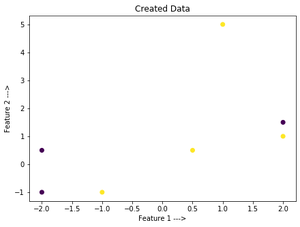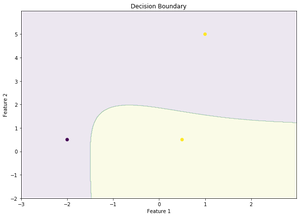# ML – Decision Function

Decision function is a method present in classifier{ SVC, Logistic Regression } class of sklearn machine learning framework. This method basically returns a Numpy array, In which each element represents whether a predicted sample for x_test by the classifier lies to the right or left side of the Hyperplane and also how far from the HyperPlane.

It also tells us that how confidently each value predicted for x_test by the classifier is Positive ( large-magnitude Positive value ) or Negative ( large-magnitude Negative value).

Math behind the Decision Function method:
Let’s consider the SVM for linearly-separable binary class classification problem:

Cost Function:Hypothesis for this Linearly Separable Binary class classification:The optimization Algorithm minimizes the cost function to find the best value of the model parameter for the hypothesis such that:What Actually happens when we pass a data instance to Decision Function method ?
This data sample is substituted in this hypothesis whose model parameters have been found by minimizing the cost function and returns the value outputted by this hypothesis which would be >1 if actual output is 1 or <-1 if the actual output is 0. This returned value indeed represents on which side of the hyperplane and also how far from it the given data sample lie.

Code: create our own data set and plot the input.

 # This code may not run on GFG IDE  # As required modules are not available.     # Create a simple data set  # Binary-Class Classification.     # Import Required Modules.  import matplotlib.pyplot as plt  import numpy as np     # Input Feature X.  x = np.array([[2, 1.5], [-2, -1], [-1, -1], [2, 1],                [1, 5], [0.5, 0.5], [-2, 0.5]])     # Input Feature Y.  y = np.array([0, 0, 1, 1, 1, 1, 0])     # Training set Featute x_train.  x_train = np.array([[2, 1.5], [-2, -1], [-1, -1], [2, 1]])     # Training set Target Variable y_train.  y_train = np.array([0, 0, 1, 1])     # Test set Featute x_test.  x_test = np.array([[1, 5], [0.5, 0.5], [-2, 0.5]])     # Test set Target Variable y_test  y_test = np.array([1, 1, 0])     # Plot the obtained data  plt.scatter(x[:, 0], x[:, 1], c = y)  plt.xlabel('Feature 1 --->')  plt.ylabel('Feature 2 --->')  plt.title('Created Data')

Output:Code: train our model

 # This code may not run on GFG IDE  # As required modules are not available.     # Import SVM Class from sklearn.  from sklearn.svm import SVC  clf = SVC()     # Train the model on the training set.  clf.fit(x_train, y_train)      # Predict on Test set  predict = clf.predict(x_test)  print('Predicted Values from Classifier:', predict)  print('Actual Output is:', y_test)  print('Accuracy of the model is:', clf.score(x_test, y_test))

Output:

Predicted Values from Classifier: [0 1 0]
Actual Output is: [1 1 0]
Accuracy of the model is: 0.6666666666666666


Code: decision function method

 # This code may not run on GFG IDE  # As required modules are not available.     # Using Decision Function Method Present in svc class  Decision_Function = clf.decision_function(x_test)  print('Output of Decision Function is:', Decision_Function)  print('Prediction for x_test from classifier is:', predict)

Output:

Output of Decision Function is: [-0.04274893  0.29143233 -0.13001369]
Prediction for x_test from classifier is: [0 1 0]


From the above output, we can conclude that the decision function output represents whether a predicted sample for x_test by the classifier lies to the right side or left side of hyperplane and also how far from it. It also tells us how confidently each value predicted for x_test by the classifier is Positive ( large-magnitude Positive value ) or Negative ( large-magnitude Negative value)

Code: Decision Boundary

 # This code may not run on GFG IDE  # As required modules are not available.     # To Plot the Decision Boundary.  arr1 = np.arange(x[:, 0].min()-1, x[:, 0].max()+1, 0.01)  arr2 = np.arange(x[:, 1].min()-1, x[:, 1].max()+1, 0.01)     xx, yy = np.meshgrid(arr1, arr2)  input_array = np.array([xx.ravel(), yy.ravel()]).T  labels = clf.predict(input_array)     plt.figure(figsize =(10, 7))  plt.contourf(xx, yy, labels.reshape(xx.shape), alpha = 0.1)  plt.scatter(x_test[:, 0], x_test[:, 1], c = y_test.ravel(), alpha = 1)  plt.xlabel('Feature 1')  plt.ylabel('Feature 2')  plt.title('Decision Boundary')

Let’s Visualize the above conclusion.The advantage of Decision Function output is to set DECISION THRESHOLD and predict a new output for x_test, such that we get desired precision or recall value If our project is precision-oriented or recall-oriented respectively.

Attention geek! Strengthen your foundations with the Python Programming Foundation Course and learn the basics.

To begin with, your interview preparations Enhance your Data Structures concepts with the Python DS Course.

My Personal Notes arrow_drop_upCheck out this Author's contributed articles.

If you like GeeksforGeeks and would like to contribute, you can also write an article using contribute.geeksforgeeks.org or mail your article to contribute@geeksforgeeks.org. See your article appearing on the GeeksforGeeks main page and help other Geeks.

Please Improve this article if you find anything incorrect by clicking on the "Improve Article" button below.

Article Tags :
Practice Tags :

2

Please write to us at contribute@geeksforgeeks.org to report any issue with the above content.